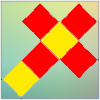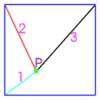Search by Topic

Resources tagged with Pythagoras' theorem similar to Equation Attack:

Filter by: Content type:
Age range:
Challenge level:

There are 79 results

Broad Topics > Pythagoras and Trigonometry > Pythagoras' theoremFar Horizon

Age 14 to 16 Challenge Level:

An observer is on top of a lighthouse. How far from the foot of the lighthouse is the horizon that the observer can see?Spherical Triangles on Very Big Spheres

Age 16 to 18 Challenge Level:

Shows that Pythagoras for Spherical Triangles reduces to Pythagoras's Theorem in the plane when the triangles are small relative to the radius of the sphere.Chord

Age 16 to 18 Challenge Level:

Equal touching circles have centres on a line. From a point of this line on a circle, a tangent is drawn to the farthest circle. Find the lengths of chords where the line cuts the other circles.Where to Land

Age 14 to 16 Challenge Level:

Chris is enjoying a swim but needs to get back for lunch. If she can swim at 3 m/s and run at 7m/sec, how far along the bank should she land in order to get back as quickly as possible?Slippage

Age 14 to 16 Challenge Level:

A ladder 3m long rests against a wall with one end a short distance from its base. Between the wall and the base of a ladder is a garden storage box 1m tall and 1m high. What is the maximum distance. . . .Under the Ribbon

Age 14 to 16 Challenge Level:

A ribbon is nailed down with a small amount of slack. What is the largest cube that can pass under the ribbon ?Cubestick

Age 16 to 18 Challenge Level:

Stick some cubes together to make a cuboid. Find two of the angles by as many different methods as you can devise.Nicely Similar

Age 14 to 16 Challenge Level:

If the hypotenuse (base) length is 100cm and if an extra line splits the base into 36cm and 64cm parts, what were the side lengths for the original right-angled triangle?Pythagoras for a Tetrahedron

Age 16 to 18 Challenge Level:

In a right-angled tetrahedron prove that the sum of the squares of the areas of the 3 faces in mutually perpendicular planes equals the square of the area of the sloping face. A generalisation. . . .Squ-areas

Age 14 to 16 Challenge Level:

Three squares are drawn on the sides of a triangle ABC. Their areas are respectively 18 000, 20 000 and 26 000 square centimetres. If the outer vertices of the squares are joined, three more. . . .Compare Areas

Age 14 to 16 Challenge Level:

Which has the greatest area, a circle or a square inscribed in an isosceles, right angle triangle?Baby Circle

Age 16 to 18 Challenge Level:

A small circle fits between two touching circles so that all three circles touch each other and have a common tangent? What is the exact radius of the smallest circle?Age 14 to 16 Challenge Level:

A 1 metre cube has one face on the ground and one face against a wall. A 4 metre ladder leans against the wall and just touches the cube. How high is the top of the ladder above the ground?Fitting In

Age 14 to 16 Challenge Level:

The largest square which fits into a circle is ABCD and EFGH is a square with G and H on the line CD and E and F on the circumference of the circle. Show that AB = 5EF. Similarly the largest. . . .Strange Rectangle

Age 16 to 18 Challenge Level:

ABCD is a rectangle and P, Q, R and S are moveable points on the edges dividing the edges in certain ratios. Strangely PQRS is always a cyclic quadrilateral and you can find the angles.Are You Kidding

Age 14 to 16 Challenge Level:

If the altitude of an isosceles triangle is 8 units and the perimeter of the triangle is 32 units.... What is the area of the triangle?Retracircles

Age 16 to 18 Challenge Level:

Four circles all touch each other and a circumscribing circle. Find the ratios of the radii and prove that joining 3 centres gives a 3-4-5 triangle.Two Circles

Age 14 to 16 Challenge Level:

Draw two circles, each of radius 1 unit, so that each circle goes through the centre of the other one. What is the area of the overlap?Matter of Scale

Age 14 to 16 Challenge Level:

Prove Pythagoras' Theorem using enlargements and scale factors.All Tied Up

Age 14 to 16 Challenge Level:

A ribbon runs around a box so that it makes a complete loop with two parallel pieces of ribbon on the top. How long will the ribbon be?Classic Cube

Age 16 to 18 Challenge Level:

The net of a cube is to be cut from a sheet of card 100 cm square. What is the maximum volume cube that can be made from a single piece of card?Golden Construction

Age 16 to 18 Challenge Level:

Draw a square and an arc of a circle and construct the Golden rectangle. Find the value of the Golden Ratio.The Fire-fighter's Car Keys

Age 14 to 16 Challenge Level:

A fire-fighter needs to fill a bucket of water from the river and take it to a fire. What is the best point on the river bank for the fire-fighter to fill the bucket ?.Partly Circles

Age 14 to 16 Challenge Level:

What is the same and what is different about these circle questions? What connections can you make?Pythagoras Proofs

Age 14 to 16 Challenge Level:

Can you make sense of these three proofs of Pythagoras' Theorem?Square Pair Circles

Age 16 to 18 Challenge Level:

Investigate the number of points with integer coordinates on circles with centres at the origin for which the square of the radius is a power of 5.Kite in a Square

Age 14 to 16 Challenge Level:

Can you make sense of the three methods to work out the area of the kite in the square?Square World

Age 16 to 18 Challenge Level:

P is a point inside a square ABCD such that PA= 1, PB = 2 and PC = 3. How big is angle APB ?Orthogonal Circle

Age 16 to 18 Challenge Level:

Given any three non intersecting circles in the plane find another circle or straight line which cuts all three circles orthogonally.Ball Packing

Age 14 to 16 Challenge Level:

If a ball is rolled into the corner of a room how far is its centre from the corner?Rectangular Pyramids

Age 14 to 18 Challenge Level:

Is the sum of the squares of two opposite sloping edges of a rectangular based pyramid equal to the sum of the squares of the other two sloping edges?Medallions

Age 14 to 16 Challenge Level:

Three circular medallions fit in a rectangular box. Can you find the radius of the largest one?Three Four Five

Age 14 to 16 Challenge Level:

Two semi-circles (each of radius 1/2) touch each other, and a semi-circle of radius 1 touches both of them. Find the radius of the circle which touches all three semi-circles.At a Glance

Age 14 to 16 Challenge Level:

The area of a regular pentagon looks about twice as a big as the pentangle star drawn within it. Is it?Napkin

Age 14 to 16 Challenge Level:

A napkin is folded so that a corner coincides with the midpoint of an opposite edge . Investigate the three triangles formed .Semi-detached

Age 14 to 16 Challenge Level:

A square of area 40 square cms is inscribed in a semicircle. Find the area of the square that could be inscribed in a circle of the same radius.Inscribed in a Circle

Age 14 to 16 Challenge Level:

The area of a square inscribed in a circle with a unit radius is, satisfyingly, 2. What is the area of a regular hexagon inscribed in a circle with a unit radius?Rhombus in Rectangle

Age 14 to 16 Challenge Level:

Take any rectangle ABCD such that AB > BC. The point P is on AB and Q is on CD. Show that there is exactly one position of P and Q such that APCQ is a rhombus.Grid Lockout

Age 14 to 16 Challenge Level:

What remainders do you get when square numbers are divided by 4?Holly

Age 14 to 16 Challenge Level:

The ten arcs forming the edges of the "holly leaf" are all arcs of circles of radius 1 cm. Find the length of the perimeter of the holly leaf and the area of its surface.Generating Triples

Age 14 to 16 Challenge Level:

Sets of integers like 3, 4, 5 are called Pythagorean Triples, because they could be the lengths of the sides of a right-angled triangle. Can you find any more?Circle Scaling

Age 14 to 16 Challenge Level:

Describe how to construct three circles which have areas in the ratio 1:2:3.Circle Box

Age 14 to 16 Challenge Level:

It is obvious that we can fit four circles of diameter 1 unit in a square of side 2 without overlapping. What is the smallest square into which we can fit 3 circles of diameter 1 unit?The Spider and the Fly

Age 14 to 16 Challenge Level:

A spider is sitting in the middle of one of the smallest walls in a room and a fly is resting beside the window. What is the shortest distance the spider would have to crawl to catch the fly?Corridors

Age 14 to 16 Challenge Level:

A 10x10x10 cube is made from 27 2x2 cubes with corridors between them. Find the shortest route from one corner to the opposite corner.Take a Square

Age 14 to 16 Challenge Level:

Cut off three right angled isosceles triangles to produce a pentagon. With two lines, cut the pentagon into three parts which can be rearranged into another square.Incircles

Age 16 to 18 Challenge Level:

The incircles of 3, 4, 5 and of 5, 12, 13 right angled triangles have radii 1 and 2 units respectively. What about triangles with an inradius of 3, 4 or 5 or ...?Six Discs

Age 14 to 16 Challenge Level:

Six circular discs are packed in different-shaped boxes so that the discs touch their neighbours and the sides of the box. Can you put the boxes in order according to the areas of their bases?